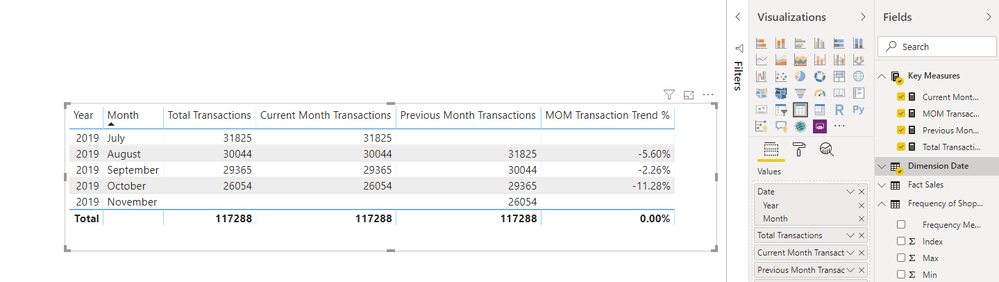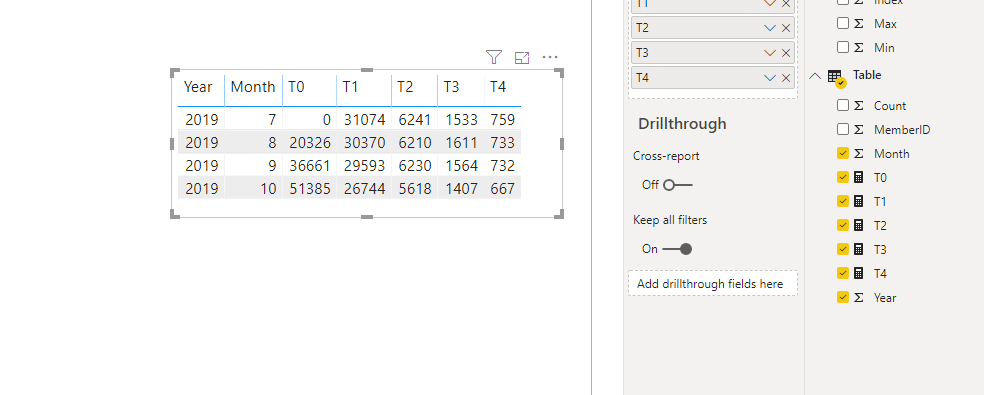cancel
Showing results for
Did you mean:

Fabric is Generally Available. Browse Fabric Presentations. Work towards your Fabric certification with the Cloud Skills Challenge.Helper I

## Purchase Frequency - Customers with 0, 1,2,3,4 or more transactions for current month

Hello,

I am having trouble with frequency calculations.I need to create a table counting the number of customers with 0 transactions or 1 or 2 or 3 for the current month, the previous month and include a month over month % trend. I created a table which includes the metrics i must report on but cant get my calculations correct, or link them to my created table. The exisiting tables i have are Dimension Date and Fact Sales. Below are some of the Dax calculations im using:

Total Transactions = DISTINCTCOUNT('Fact Sales'[ID])
Current Month Transactions = CALCULATE([Total Transactions],FILTER('Fact Sales',MONTH('Fact Sales'[TransactionDateTime].[Date])=MONTH(TODAY())))
Previous Month Transactions = var current_month= MONTH(TODAY()) return
CALCULATE([Total Transactions],FILTER('Fact Sales',MONTH('Fact Sales'[transactiondatetime].[date])=current_month -1))

Any help would be greatly appreciated 🙂

1 ACCEPTED SOLUTIONCommunity Support

HI @ClaireBear ,

I modify your formulas and it can display the current and previous transactions count based on dimension date.

``````Current Month Transactions =
CALCULATE (
[Total Transactions],
FILTER (
ALLSELECTED ( 'Fact Sales' ),
DATEVALUE ( 'Fact Sales'[TransactionDateTime] )
IN VALUES ( 'Dimension Date'[Date] )
)
)

Previous Month Transactions =
CALCULATE (
[Total Transactions],
FILTER (
ALLSELECTED ( 'Fact Sales' ),
DATEVALUE ( 'Fact Sales'[transactiondatetime] )
IN DATEADD ( VALUES ( 'Dimension Date'[Date] ), -1, MONTH )
)
)

MOM Transaction Trend % =
VAR result =
DIVIDE ( [Current Month Transactions], [Previous Month Transactions], BLANK () )
RETURN
IF ( result <> BLANK (), result - 1 )
``````For transaction group, you can refer to the following steps:

1. Create a summarize table with date, member id, count.

``````Table =
SUMMARIZE (
'Fact Sales',
"Year", YEAR ( [TransactionDateTime] ),
"Month", MONTH ( [TransactionDateTime] )
),
[Year],
[Month],
[MemberID],
"Count", COUNTROWS(VALUES( ( 'Fact Sales'[TransactionID] )))
)
``````

2. Write measures to matched members based on the current date.

``````T0 =
VAR _current =
MAX ( 'Table'[Year] ) * 100
+ MAX ( 'Table'[Month] )
VAR _prevMember =
CALCULATETABLE (
VALUES ( 'Table'[MemberID] ),
FILTER ( ALL('Table'), [Year] * 100 + [Month] < _current )
)
VAR _currMember =
CALCULATETABLE (
VALUES ( 'Table'[MemberID] ),
FILTER ( 'Table', [Year] * 100 + [Month] = _current )
)
RETURN
COUNTROWS ( EXCEPT ( _prevMember,_currMember  ) )+0

T1 =
VAR _current =
MAX ( 'Table'[Year] ) * 100
+ MAX ( 'Table'[Month] )
VAR _currMember =
CALCULATETABLE (
VALUES ( 'Table'[MemberID] ),
FILTER ( 'Table', [Year] * 100 + [Month] = _current )
)
RETURN
COUNTROWS (
FILTER ( ALLSELECTED ( 'Table' ), [MemberID] IN _currMember && [Count] = 1 )
)

T2 =
VAR _current =
MAX ( 'Table'[Year] ) * 100
+ MAX ( 'Table'[Month] )
VAR _currMember =
CALCULATETABLE (
VALUES ( 'Table'[MemberID] ),
FILTER ( 'Table', [Year] * 100 + [Month] = _current )
)
RETURN
COUNTROWS (
FILTER ( ALLSELECTED ( 'Table' ), [MemberID] IN _currMember && [Count] = 2 )
)

T3 =
VAR _current =
MAX ( 'Table'[Year] ) * 100
+ MAX ( 'Table'[Month] )
VAR _currMember =
CALCULATETABLE (
VALUES ( 'Table'[MemberID] ),
FILTER ( 'Table', [Year] * 100 + [Month] = _current )
)
RETURN
COUNTROWS (
FILTER ( ALLSELECTED ( 'Table' ), [MemberID] IN _currMember && [Count] = 3 )
)
T4 =
VAR _current =
MAX ( 'Table'[Year] ) * 100
+ MAX ( 'Table'[Month] )
VAR _currMember =
CALCULATETABLE (
VALUES ( 'Table'[MemberID] ),
FILTER ( 'Table', [Year] * 100 + [Month] = _current )
)
RETURN
COUNTROWS (
FILTER ( ALLSELECTED ( 'Table' ), [MemberID] IN _currMember && [Count] >= 4 )
)``````

3. Create a table visual with date fields and measures.Notice: I also attached the sample file below.

Regards,

Xiaoxin Sheng

Community Support Team _ Xiaoxin
If this post helps, please consider accept as solution to help other members find it more quickly.
5 REPLIES 5Community Support

HI @ClaireBear ,

I modify your formulas and it can display the current and previous transactions count based on dimension date.

``````Current Month Transactions =
CALCULATE (
[Total Transactions],
FILTER (
ALLSELECTED ( 'Fact Sales' ),
DATEVALUE ( 'Fact Sales'[TransactionDateTime] )
IN VALUES ( 'Dimension Date'[Date] )
)
)

Previous Month Transactions =
CALCULATE (
[Total Transactions],
FILTER (
ALLSELECTED ( 'Fact Sales' ),
DATEVALUE ( 'Fact Sales'[transactiondatetime] )
IN DATEADD ( VALUES ( 'Dimension Date'[Date] ), -1, MONTH )
)
)

MOM Transaction Trend % =
VAR result =
DIVIDE ( [Current Month Transactions], [Previous Month Transactions], BLANK () )
RETURN
IF ( result <> BLANK (), result - 1 )
``````For transaction group, you can refer to the following steps:

1. Create a summarize table with date, member id, count.

``````Table =
SUMMARIZE (
'Fact Sales',
"Year", YEAR ( [TransactionDateTime] ),
"Month", MONTH ( [TransactionDateTime] )
),
[Year],
[Month],
[MemberID],
"Count", COUNTROWS(VALUES( ( 'Fact Sales'[TransactionID] )))
)
``````

2. Write measures to matched members based on the current date.

``````T0 =
VAR _current =
MAX ( 'Table'[Year] ) * 100
+ MAX ( 'Table'[Month] )
VAR _prevMember =
CALCULATETABLE (
VALUES ( 'Table'[MemberID] ),
FILTER ( ALL('Table'), [Year] * 100 + [Month] < _current )
)
VAR _currMember =
CALCULATETABLE (
VALUES ( 'Table'[MemberID] ),
FILTER ( 'Table', [Year] * 100 + [Month] = _current )
)
RETURN
COUNTROWS ( EXCEPT ( _prevMember,_currMember  ) )+0

T1 =
VAR _current =
MAX ( 'Table'[Year] ) * 100
+ MAX ( 'Table'[Month] )
VAR _currMember =
CALCULATETABLE (
VALUES ( 'Table'[MemberID] ),
FILTER ( 'Table', [Year] * 100 + [Month] = _current )
)
RETURN
COUNTROWS (
FILTER ( ALLSELECTED ( 'Table' ), [MemberID] IN _currMember && [Count] = 1 )
)

T2 =
VAR _current =
MAX ( 'Table'[Year] ) * 100
+ MAX ( 'Table'[Month] )
VAR _currMember =
CALCULATETABLE (
VALUES ( 'Table'[MemberID] ),
FILTER ( 'Table', [Year] * 100 + [Month] = _current )
)
RETURN
COUNTROWS (
FILTER ( ALLSELECTED ( 'Table' ), [MemberID] IN _currMember && [Count] = 2 )
)

T3 =
VAR _current =
MAX ( 'Table'[Year] ) * 100
+ MAX ( 'Table'[Month] )
VAR _currMember =
CALCULATETABLE (
VALUES ( 'Table'[MemberID] ),
FILTER ( 'Table', [Year] * 100 + [Month] = _current )
)
RETURN
COUNTROWS (
FILTER ( ALLSELECTED ( 'Table' ), [MemberID] IN _currMember && [Count] = 3 )
)
T4 =
VAR _current =
MAX ( 'Table'[Year] ) * 100
+ MAX ( 'Table'[Month] )
VAR _currMember =
CALCULATETABLE (
VALUES ( 'Table'[MemberID] ),
FILTER ( 'Table', [Year] * 100 + [Month] = _current )
)
RETURN
COUNTROWS (
FILTER ( ALLSELECTED ( 'Table' ), [MemberID] IN _currMember && [Count] >= 4 )
)``````

3. Create a table visual with date fields and measures.Notice: I also attached the sample file below.

Regards,

Xiaoxin Sheng

Community Support Team _ Xiaoxin
If this post helps, please consider accept as solution to help other members find it more quickly.Anonymous
Not applicable

Current Month Transactions = CALCULATE(DISTINCTCOUNT('Fact Sales'[ID]),PARALLELPERIOD('Fact Sales'[TransactionDateTime],0,MONTH))
Previous Month Transactions = CALCULATE(DISTINCTCOUNT('Fact Sales'[ID]),PREVIOUSMONTH('Fact Sales'[transactiondatetime]))Helper I

Hi  Icespedes,

i tried your suggestion and unfortunately this is not working, i need to link the table i created (transaction frequency) to the measures by current month, previous month and % change. This is to calculate what percentage of total customers had 0 transactions for the current month, how many had 0 transactions for the previous month and what is the difference. Then, how many customers had only 1 transaction for the current month, how many customers had 1 transaction for the previous month and the difference etc etc.  I need to represent this in a table format.ThanksCommunity Support

HI @ClaireBear ,

Can you please share some sample data for test? It is hard to test and coding formula from your snapshot.

Regards,

Xiaoxin Sheng

Community Support Team _ Xiaoxin
If this post helps, please consider accept as solution to help other members find it more quickly.Helper I

Thank you so much Xiaoxin.

I have uploaded a pbix file with test data to OneDrive:

Test Data.PBIX

I somehow need to link the frequency metric to the dax calculations in the same table.Announcements#### Power BI Monthly Update - November 2023

Check out the November 2023 Power BI update to learn about new features.#### Fabric Community News unified experience

Read the latest Fabric Community announcements, including updates on Power BI, Synapse, Data Factory and Data Activator.#### The largest Power BI and Fabric virtual conference

130+ sessions, 130+ speakers, Product managers, MVPs, and experts. All about Power BI and Fabric. Attend online or watch the recordings.Top Solution Authors
Top Kudoed Authors
Users online (4,747)